The UCM Procedure

SPLINEREG Statement

• SPLINEREG regressor <options> ;

The SPLINEREG statement is used to specify a regressor that has a nonlinear relationship with the dependent series that can be approximated by a given B-spline. If the specified spline has degree d and is based on n internal knots, then it is known that it can be written as a linear combination of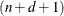regressors that are derived from the original regressor. The span of thesederived regressors includes constant; therefore, to avoid multicollinearity with the level component, one of these regressors is dropped. Specifying the SPLINEREG statement is equivalent to specifying a RANDOMREG statement with these derived regressors. There can be multiple SPLINEREG statements. You must specify at least one interior knot, either using the NKNOTS= option or the KNOTS= option. For additional information about splines, see Chapter 104: The TRANSREG Procedure in SAS/STAT 13.2 User's Guide. For an example of using this statement, see Example 34.6. See the section Reporting Parameter Estimates for Random Regressors for additional information about the way parameter estimates are reported for this type of regressors.

DEGREE=integer

specifies the degree of the spline. It can be any integer larger than or equal to zero. The default value is 3. The polynomial degree should be a small integer, usually 0, 1, 2, or 3. Larger values are rarely useful. If you have any doubt as to what degree to specify, use the default.

KNOTS=number-list |  n TO m BY p

specifies the interior knots or break points. The values in the knot list must be nondecreasing and must lie between the minimum and the maximum of the spline regressor values in the input data set. The first time you specify a value in the knot list, it indicates a discontinuity in the nth (from DEGREE=n) derivative of the transformation function at the value of the knot. The second mention of a value indicates a discontinuity in the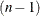th derivative of the transformation function at the value of the knot. Knots can be repeated any number of times for decreasing smoothness at the break points, but the values in the knot list can never decrease.

You cannot use the KNOTS= option with the NKNOTS= option. You should keep the number of knots small.

NKNOTS=m

creates m knots, the first at the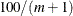percentile, the second at the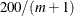percentile, and so on. Knots are always placed at data values; there is no interpolation. For example, if NKNOTS=3, knots are placed at the 25th percentile, the median, and the 75th percentile. The value specified for the NKNOTS= option must be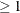. You cannot use the NKNOTS= option with the KNOTS= option.

Note: Specifying knots by using the NKNOTS= option can result in different sets of knots in the estimation and forecast stages if the distributions of regressor values in the estimation and forecast spans differ. The estimation span is based on the BACK= and SKIPFIRST= options in the ESTIMATE statement, and the forecast span is based on the BACK= and SKIPFIRST= options in the FORECAST statement.

NOEST

fixes the value of the regression coefficient random walk disturbance variance to the value specified in the VARIANCE= option.

PLOT=FILTER
PLOT=SMOOTH
PLOT=( <FILTER> <SMOOTH> )

requests plotting of filtered or smoothed estimate of the time-varying regression coefficient.

PRINT=FILTER
PRINT=SMOOTH
PRINT=( <FILTER> <SMOOTH> )

requests printing of filtered or smoothed estimate of the time-varying regression coefficient.

VARIANCE=value

specifies an initial value for the regression coefficient random walk disturbance variance during the parameter estimation process. Any nonnegative value, including zero, is an acceptable starting value.